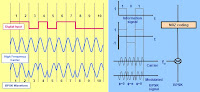# How QPSK & BPSK Modulation in WCDMA?

Posted in WCDMA
at 2016.06.20A data-modulation scheme defines how the data bits are mixed with the carrier signal, which is always a sine wave. There are three basic ways to modulate a carrier signal in a digital sense: amplitude shift keying (ASK), frequency shift keying (FSK), and phase shift keying (PSK).
• In ASK the amplitude of the carrier signal is modified by the digital signal.
• In FSK the frequency of the carrier signal is modified by the digital signal.
• The PSK family is the most widely used modulation scheme in modern cellular systems.

Digital Modulation – BPSKIn binary phase shift keying (BPSK) modulation, each data bit is transformed into a separate data symbol. The mapping rule is 1 ?> + 1 and 0 ? > ? 1. There are only two possible phase shifts in BPSK, 0 and ? radians. NRZ means none return zero.

Digital Modulation – QPSKThe quadrature phase shift keying (QPSK) modulation has four phases: 0, ?/2, ?, and 3?/2 radians. Two data bits are transformed into one complex data symbol; A symbol is any change (keying) of the carrier.

Article Topics :

ask, bit, carrier, cdma, cell, digital, fsk, modulation, phase, psk, signal, telecom, where bpsk modulation scheme are used